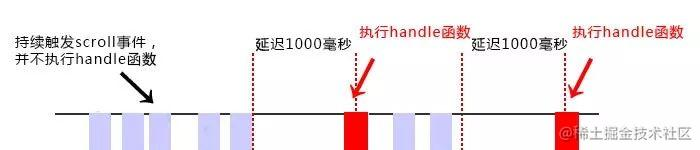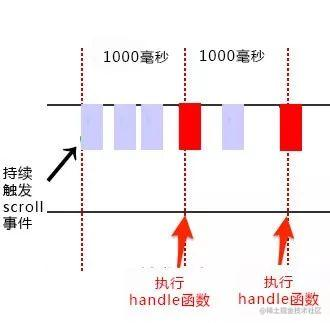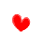# JS的防抖与节流``````function debounce(fn, wait) {
var timeout = null;
return function() {
if(timeout !== null)
clearTimeout(timeout);
timeout = setTimeout(fn, wait);
}
}
// 处理函数
function handle() {
console.log(Math.random());
}
// 滚动事件``````var throttle = function(func, delay) {
var prev = Date.now();
return function() {
var context = this;
var args = arguments;
var now = Date.now();
if (now - prev >= delay) {
func.apply(context, args);
prev = Date.now();
}
}
}
function handle() {
console.log(Math.random());
}`````` var throttle = function(func, delay) {
var timer = null;
return function() {
var context = this;
var args = arguments;
if (!timer) {
timer = setTimeout(function() {
func.apply(context, args);
timer = null;
}, delay);
}
}
}
function handle() {
console.log(Math.random());
}

``````var throttle = function(func, delay) {
var timer = null;
var startTime = Date.now();
return function() {
var curTime = Date.now();
var remaining = delay - (curTime - startTime);
var context = this;
var args = arguments;
clearTimeout(timer);
if (remaining <= 0) {
func.apply(context, args);
startTime = Date.now();
} else {
timer = setTimeout(func, remaining);
}
}
}
function handle() {
console.log(Math.random());
}唔~感谢阅读！能力有限，自我感觉写的不是特别好，欢迎小伙伴们指正交流。(*^▽^*)